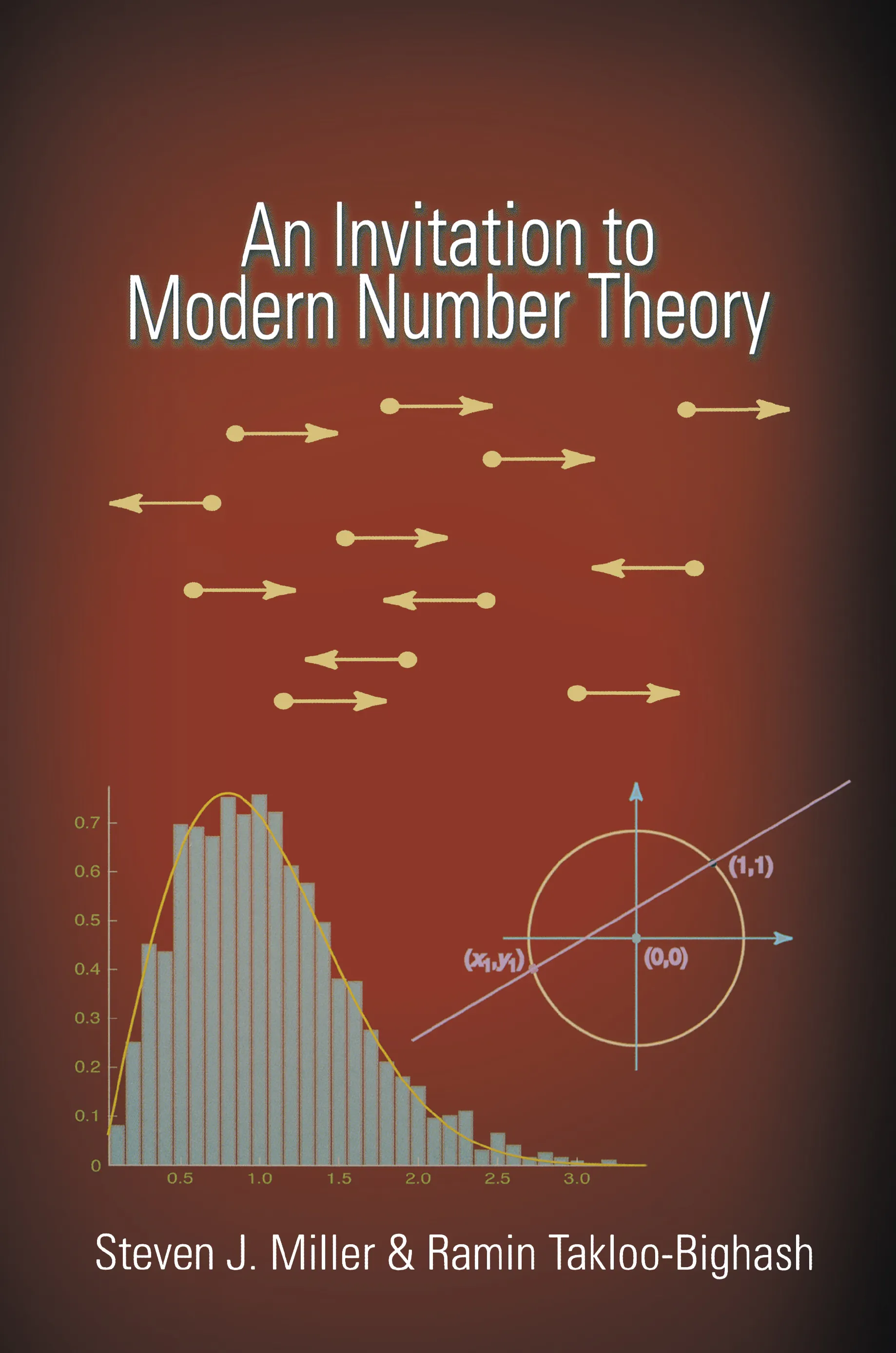Steven J. Miller

# An Invitation to Modern Number Theory### Hardcover

Price:
\$99.95 / £82.00
ISBN:
Published:
03/26/2006
2006
Pages:
528
Size:
6 x 9.25 in.
Illus:
20 line illus.

In a manner accessible to beginning undergraduates, An Invitation to Modern Number Theory introduces many of the central problems, conjectures, results, and techniques of the field, such as the Riemann Hypothesis, Roth’s Theorem, the Circle Method, and Random Matrix Theory. Showing how experiments are used to test conjectures and prove theorems, the book allows students to do original work on such problems, often using little more than calculus (though there are numerous remarks for those with deeper backgrounds). It shows students what number theory theorems are used for and what led to them and suggests problems for further research.

Steven Miller and Ramin Takloo-Bighash introduce the problems and the computational skills required to numerically investigate them, providing background material (from probability to statistics to Fourier analysis) whenever necessary. They guide students through a variety of problems, ranging from basic number theory, cryptography, and Goldbach’s Problem, to the algebraic structures of numbers and continued fractions, showing connections between these subjects and encouraging students to study them further. In addition, this is the first undergraduate book to explore Random Matrix Theory, which has recently become a powerful tool for predicting answers in number theory.

Providing exercises, references to the background literature, and Web links to previous student research projects, An Invitation to Modern Number Theory can be used to teach a research seminar or a lecture class.